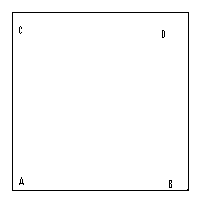### Square CalculatorCalculate the various properties of square like area, perimeter and diagonal for given values. The Square has all equal sides (4-sides) and all interior angles are right-angles (90°). The square is also known as quadrilaterals. The opposite sides of square are parallel.
 Area of square: [ (side)² ] Enter the length = Area of Square =Perimeter of square: [ 4(side) ] Enter the length = Perimeter of Square =Diagonal of square: [ (side)(sqrt(2)) ] Enter the length = Diagonal of Square =

 Attribute Definition Formula Vertex The square has four vertices.The Vertex is the corner of the squareDiagonals Both diagonal of the square cuts each other into two equal parts. The each diagonal of any square is the perpendicular bisector of the other diagonal.[(side)√2] Perimeter The perimeter is the four times length of one-side means that the distance around the square. [ 4(side) ] Area The area of quadrilaterals is Length x Height means length of one side times the perpendicular height. In case of square length and height is equal therefore we can say it is side X side. [ (side)2 ]